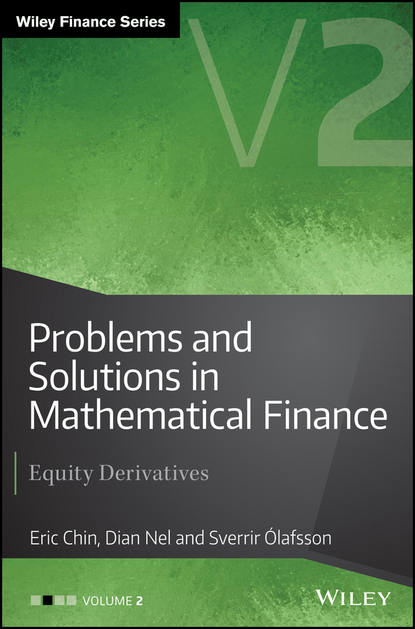﻿ Problems and Solutions in Mathematical Finance. Equity Derivatives, Volume 2 (Eric Chin) – скачать в pdf на FenzinСкачать полностью

# Problems and Solutions in Mathematical Finance. Equity Derivatives, Volume 2

PDF-книга
Добавлено 21.02.2020
Год издания: 2017
Аннотация

Detailed guidance on the mathematics behind equity derivatives Problems and Solutions in Mathematical Finance Volume II is an innovative reference for quantitative practitioners and students, providing guidance through a range of mathematical problems encountered in the finance industry. This volume focuses solely on equity derivatives problems, beginning with basic problems in derivatives securities before moving on to more advanced applications, including the construction of volatility surfaces to price exotic options. By providing a methodology for solving theoretical and practical problems, whilst explaining the limitations of financial models, this book helps readers to develop the skills they need to advance their careers. The text covers a wide range of derivatives pricing, such as European, American, Asian, Barrier and other exotic options. Extensive appendices provide a summary of important formulae from calculus, theory of probability, and differential equations, for the convenience of readers. As Volume II of the four-volume Problems and Solutions in Mathematical Finance series, this book provides clear explanation of the mathematics behind equity derivatives, in order to help readers gain a deeper understanding of their mechanics and a firmer grasp of the calculations. Review the fundamentals of equity derivatives Work through problems from basic securities to advanced exotics pricing Examine numerical methods and detailed derivations of closed-form solutions Utilise formulae for probability, differential equations, and more Mathematical finance relies on mathematical models, numerical methods, computational algorithms and simulations to make trading, hedging, and investment decisions. For the practitioners and graduate students of quantitative finance, Problems and Solutions in Mathematical Finance Volume II provides essential guidance principally towards the subject of equity derivatives.

Полная версия

## Читать онлайн

﻿
Отзывы о книге Problems and Solutions in Mathematical Finance. Equity Derivatives, Volume 2
Нет отзывов
Добавить отзыв

Чтобы добавить отзыв Вам нужно зарегистрироваться или авторизироваться